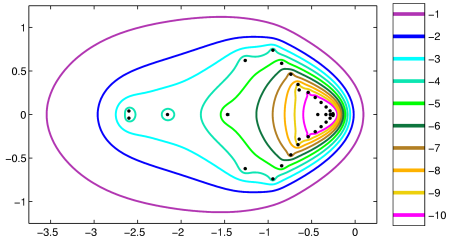Home | Introduction | Software | Examples | History | People | Bibliography | Applications | Theorems

## Pseudospectra of a Spectral Approximation of the Convection-Diffusion Operator Dimension N=39This figure illustrates approximate pseudospectra of the 1-D convection-diffusion operator u'' + u' on L2[0,d] with u(0)=u(d)=0 for d=80. The boundaries of pseudospectra of the infinite dimensional operator are shaped approximately like parabolas, as already revealed on the right part of this low-dimensional approximation. For more information, see [RT94].

Use the following MATLAB code compute a similar image using EigTool.

```  [D,x] = cheb(40);    % cheb.m from Trefethen's "Spectral Methods in MATLAB"
A = D^2/1600 + D/40;
A = A(2:end-1,2:end-1);
opts.npts=50;
opts.ax = [-3.75 0.25 -1.25 1.25];
opts.levels = -10:-1;
eigtool(A,opts)
```